# Single-Cell Array Formula in Excel

A Single-cell Array formula is powerful, yet can be hard to understand. The Evaluate Formula dialog box helps by revealing array values Excel holds in internal memory.

In the spreadsheet you see pictured, a single-cell array formula for the Cumulative Plan is located in cell B2. It summarizes the historical and current day’s Plan data. The Cumulative Actual formula, in cell C2, summarizes the Actual data in the same manner.

As an introduction to Array formulas, in my last post I added two columns. The multi-cell array formulas for columns D and E are:

{=\$A\$2>=A5:A18}

{=D5:D18*B5:B18}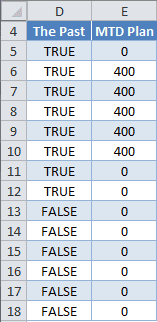Both formulas are entered by selecting their respective ranges, typing in the formula, without curly braces, and using Ctrl+Shift+Enter. Excel provides the curly braces, confirming it’s an array formula.

I didn’t include the current day in my last post, but decided to make a slight change in the column D formula here. (>= instead of >)

In the column D formula, the absolute reference to cell \$A\$2 could be replaced with the TODAY() function with the same result.

To summarize these two formulas: The Past compares Today’s date to the Date in column A and returns FALSE if the Date is in the future, and TRUE if not.

The MTD Plan multiplies this result times the Plan, and because TRUE = 1 and FALSE = 0, all rows with future date values become zero (0) and otherwise shows the value for the Plan.

## A Temporary Single-Cell Array Formula

Summarizing the MTD Plan in a single cell with an array formula will give me a temporary solution for the Cum Plan. In cell B2 I enter the array formula:

{=SUM((D5:D18)*(B5:B18))}

Again, this is done by entering =SUM((D5:D18)*(B5:B18)) and pressing Ctrl+Shift+Enter. Excel provides curly braces.

This formula multiplies together two arrays, then summarizes with the SUM function.

With a single-cell array formula, the Evaluate Formula dialog box allows me to see how this formula is being process by Excel. On the Ribbon, select the Formulas tab, then click Evaluate Formula. In Excel 2003 it’s Tools → Formula Auditing → Evaluate Formula. Excel for Mac doesn’t have this feature.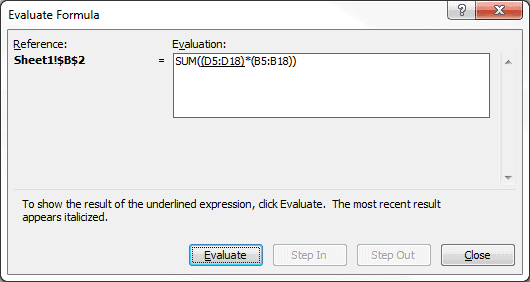To see the result of the underlined expression, click the Evaluate button. You can see below that D5:D18 is an array that Excel is holding in internal memory. The curly braces are a dead give-away for an array.The next time the Evaluate button is clicked, B5:B18 shows up as a second array with numerical values.Recall from my last post that TRUE = 1 and FALSE = 0. Multiplying these two arrays together gives a single array, which you can see by clicking the Evaluate button again.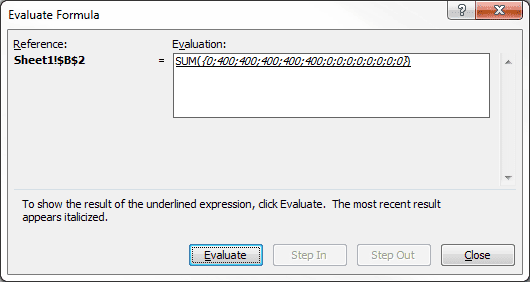The SUM function will now evaluate the array. Clicking Evaluate one more time will show the resulting answer, which is 2000.

## My Single-Cell Array Formula

I now want to get rid of columns D and E so all references to them have to be replaced. To edit the single-cell array formula for Cum Plan, I select cell B2, click inside the Formula Bar, replace D5:D18 with \$A\$2>=A5:A18, then press Ctrl+Shift+Enter to get:

{=SUM((\$A\$2>=A5:A18)*(B5:B18))}

Since cell A2 contains the TODAY() Function, the following formula works as well:

{=SUM((TODAY()>=A5:A18)*(B5:B18))}

The formula’s calculation progression is similar to the previous screen-shots of the Evaluate Formula dialog box.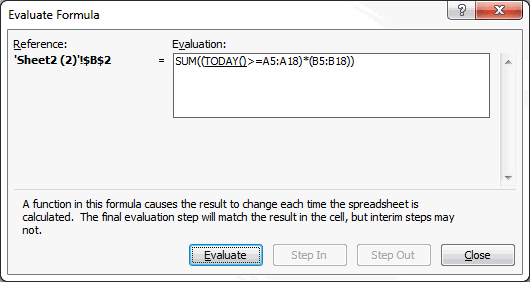The TODAY() Function evaluates to the date serial number 40490, which is November 8, 2010, and is compared to each cell in the array A5:A18 to get an array of TRUE and FALSE values. The second array B5:B18 is expanded to show the numerical values. These two arrays are multiplied together to get a single array of values, which the SUM Function then evaluates and returns the value 2000 to cell B2.

A single-cell array formula can also be applied to the Act Plan for cell C2, which is:

{=SUM((TODAY()>=A5:A18)*(C5:C18))}

And those are my single-cell array formulas.

### Note on Excel for Mac

Apparently there is no Evaluate Formula option in Excel 2008 or 2011 for Mac. I have both programs and it simply doesn’t exist. One of the help forum answers suggested using the F9 key, when editing a formula, to show the values in an array, but that doesn’t work for me. back

# Multi-Cell Array Formula in Excel

Arrays are difficult to understand because Excel holds them internally and it’s hard to conceptualize how operations are performed on the data. I had a vexing problem that was solved by using a single-cell array formula and wanted to share it with you.

However, because of the subject nature of Arrays, I’m going to show the solution to this problem in two parts. Here I’ll cover a Multi-Cell Array formula and my next post will talk about a Single-Cell Array formula, which, turns out, is my magic formula.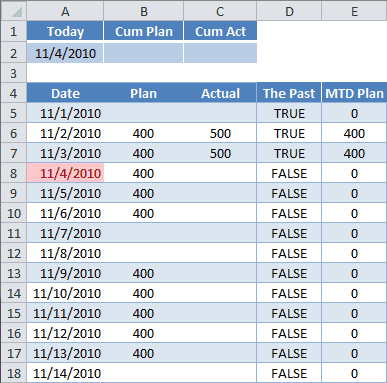### The Problem

Here’s an example worksheet. The data here is rather simple: Date, Plan, and Actual. The Plan data is set, and Actual data is plugged in when completed.

My ultimate goal is to have a formula in cell B2 for the Cum Plan, which summarizes Plan history, i.e., for all dates in the past. And keep in mind I need the formula to work with earlier versions of Excel, so no SUMIFS availability.

I’ve added two columns that will hold multi-cell array formulas, The Past and MTD Plan. But first, for reasons that will become apparent later, I’ll cover plain, regular formulas for these two columns.

#### Some Groundwork

The formula in cell D5 uses a comparison operator (>). The formula is:

=(\$A\$2>A5)

The result is TRUE. \$A\$2 is an absolute reference to cell A2, where the TODAY() Function resides and merely shows today’s computer clock date. I could have entered =TODAY()>A5 in cell D5 with the same result. When the date for Today is less than or equal to the Date in column A, we get FALSE.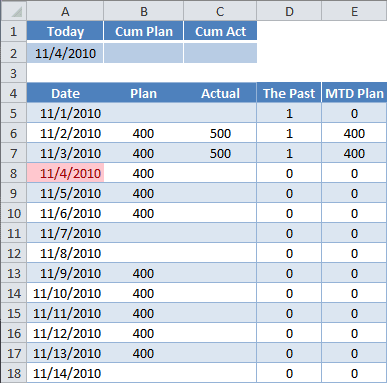TRUE and FALSE are binary values, where Excel equates TRUE = 1 and FALSE = 0. You can see this clearly by multiplying the previous formula by 1. (see picture)

=(\$A\$2>A5)*1

Any number multiplied by TRUE is like multiplying by 1, and any number multiplied by FALSE is like multiplying by zero (0).

The formula in column E for MTD Plan is to multiply The Past times Plan. The formula in cell E5 is:

=D5*B5

## Entering a Multi-Cell Array Formula

To enter any Array formula requires a special hand-shake, er, key press. You select multiple cells, type the formula, then hold the Ctrl+Shift keys down and press Enter.

The array formula in cells D5:D18 is entered by:

• Selecting cells D5:D18
• Typing the formula =\$A\$2>A5:A18
• Use keyboard combination Ctrl+Shift+Enter

Which gives you the following formula for the entire range:

{=\$A\$2>A5:A18}

The curly braces are the result of using the special keyboard combination: Ctrl+Shift+Enter. You don’t enter the curly braces, Excel does this automatically.

The array formula in cells E5:E18 is entered by:

• Selecting cells E5:E18
• Typing the formula =D5:D18*B5:B18
• Use keyboard combination Ctrl+Shift+Enter

Which gives you,

{=D5:D18*B5:B18}

in every cell of the range.

### Things to Know About a Muli-Cell Array Formula

• You must select all cells in the range before you enter the formula
• You can’t change one cell in a multi-cell array formula
• You can’t insert a row into the range of a multi-cell array formula
• To edit: select the range then press F2 (re-enter the array by Ctrl+Shift+Enter)
• To convert to a regular formula: select the range, press F2, then Ctrl+Enter
• To select the entire range: select a cell in the range, then press Ctrl+/

## Uses for a Multi-Cell Array Formula

I haven’t found the need to use a multi-cell array formula, except for a gateway to understanding a single-cell array. At least with a single-cell array you can use the Evaluate Formula dialog box to see how the Array is working in Excel’s internal memory.

Using some of the concepts I’ve introduced here, my next post will cover a Single-Cell Array Formula that solves my problem of getting one formula to show the Cumulative Plan quantity for all days before today. I promise it will be more satisfying than this post.